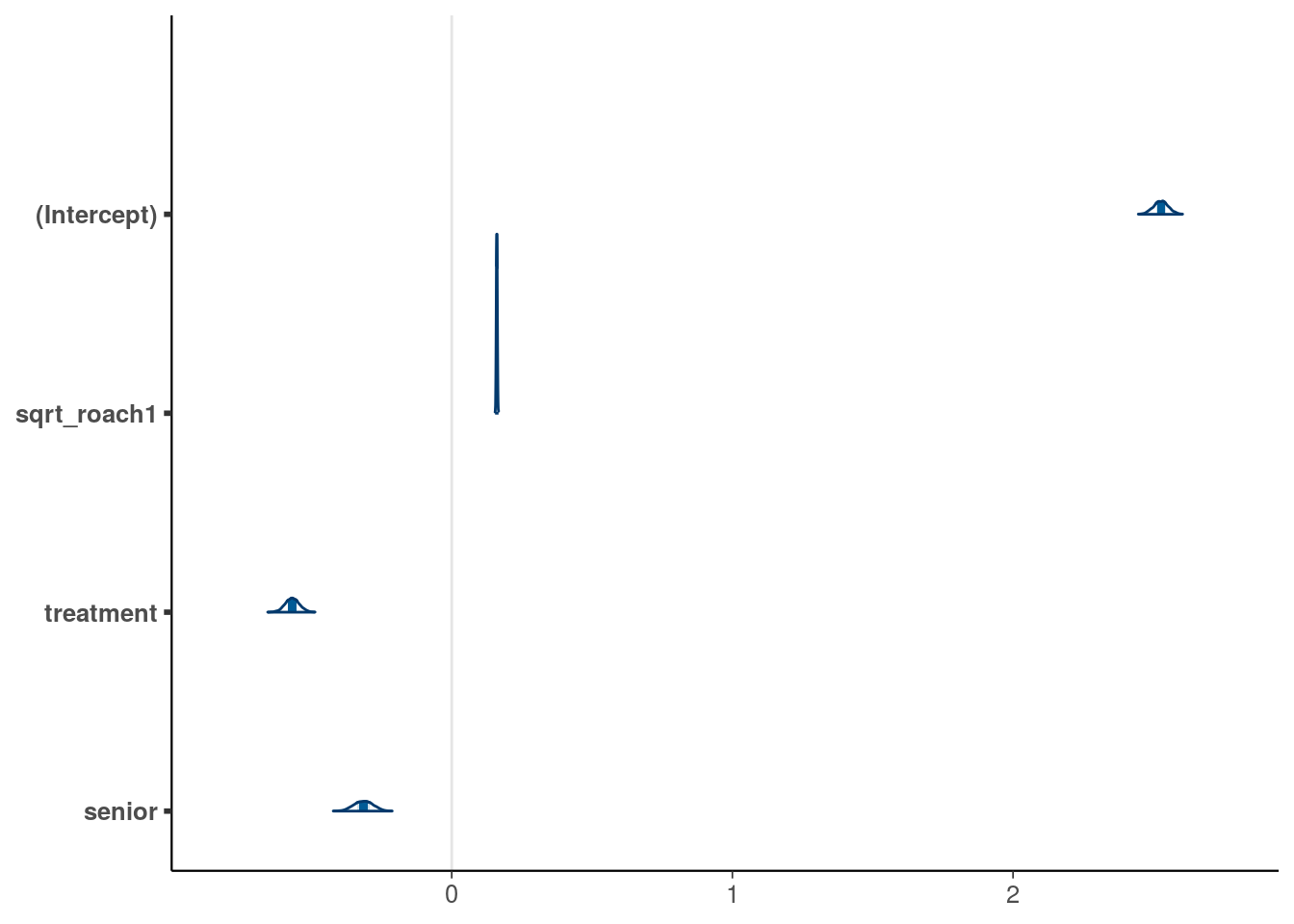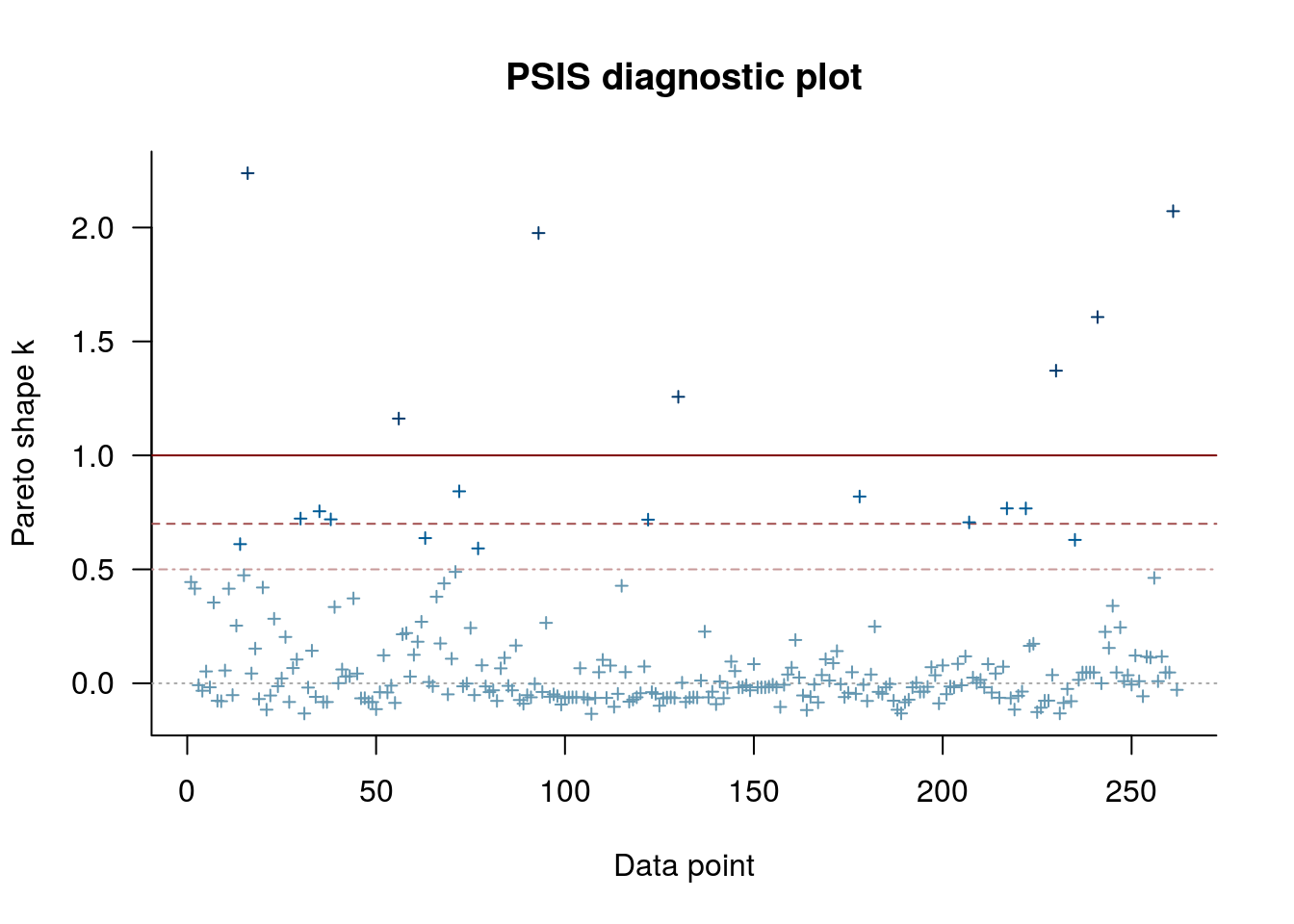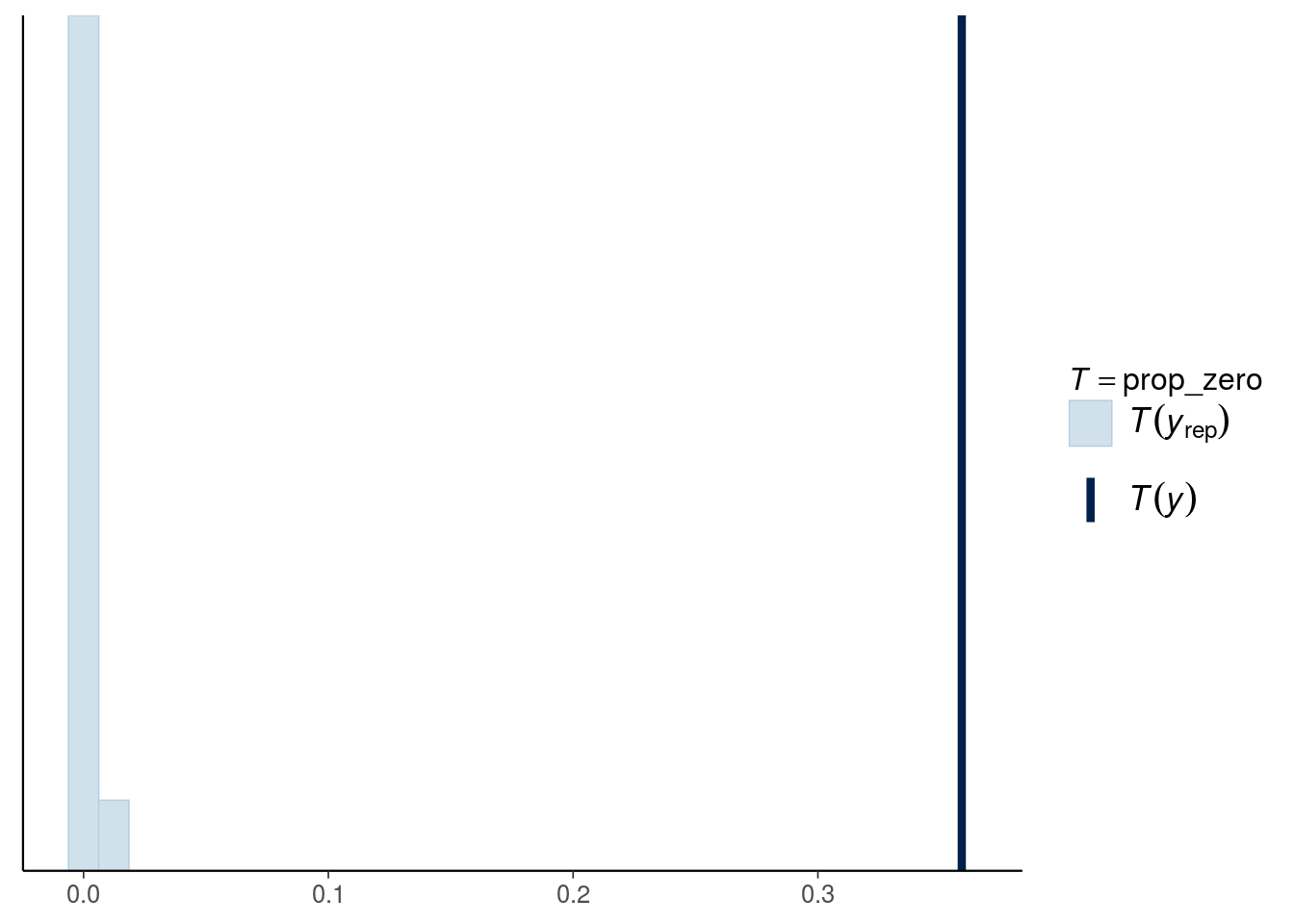# Setup

library(rstanarm)
library(brms)
options(mc.cores = parallel::detectCores())
library(loo)
library(ggplot2)
library(bayesplot)
theme_set(bayesplot::theme_default(base_family = "sans"))

# 1 Introduction

This notebook demonstrates cross-validation of simple misspecified model. In this case, cross-validation is useful to detect misspecification.

The example comes from Chapter 8.3 of Gelman and Hill (2007) and the introduction text for the data is from Estimating Generalized Linear Models for Count Data with rstanarm by Jonah Gabry and Ben Goodrich.

We want to make inferences about the efficacy of a certain pest management system at reducing the number of roaches in urban apartments. Here is how Gelman and Hill describe the experiment (pg. 161):

the treatment and control were applied to 160 and 104 apartments, respectively, and the outcome measurement $$y_i$$ in each apartment $$i$$ was the number of roaches caught in a set of traps. Different apartments had traps for different numbers of days

In addition to an intercept, the regression predictors for the model are the pre-treatment number of roaches roach1, the treatment indicator treatment, and a variable indicating whether the apartment is in a building restricted to elderly residents senior. Because the number of days for which the roach traps were used is not the same for all apartments in the sample, we include it as an exposure2 by adding $$\ln(u_i)$$) to the linear predictor $$\eta_i$$ and it can be specified using the offset argument to stan_glm.

# 2 Poisson model

data(roaches)
# Roach1 is very skewed and we take a square root
roaches$sqrt_roach1 <- sqrt(roaches$roach1)

Fit with stan_glm

stan_glmp <- stan_glm(y ~ sqrt_roach1 + treatment + senior, offset = log(exposure2),
data = roaches, family = poisson,
prior = normal(0,2.5), prior_intercept = normal(0,5),
chains = 4, cores = 1, seed = 170400963, refresh=0)

## 2.1 Analyse posterior

Plot posterior

mcmc_areas(as.matrix(stan_glmp), prob_outer = .999)We have all marginals significantly away from zero.

## 2.2 Cross-validation checking

We can use Pareto-smoothed importance sampling leave-one-out cross-validation as model checking tool (Vehtari, Gelman and Gabry, 2017).

(loop <- loo(stan_glmp))

Computed from 4000 by 262 log-likelihood matrix

Estimate     SE
elpd_loo  -5457.0  694.5
p_loo       251.5   54.3
looic     10914.1 1389.1
------
Monte Carlo SE of elpd_loo is NA.

Pareto k diagnostic values:
Count Pct.    Min. n_eff
(-Inf, 0.5]   (good)     242   92.4%   234
(0.5, 0.7]   (ok)         4    1.5%   92
(0.7, 1]   (bad)        9    3.4%   36
(1, Inf)   (very bad)   7    2.7%   1
See help('pareto-k-diagnostic') for details.

We got serious warnings, let’s plot Pareto $$k$$ values.

plot(loop)There are several observations which are highly influential, which indicates potential model misspecification (Vehtari, Gelman and Gabry, 2017).

Before looking in more detail where the problem is or fixing it, let’s check what would cross-validation say about relevance of covariates.

We form 3 models by dropping each of the covariates out.

stan_glmm1p <- update(stan_glmp, formula = y ~ treatment + senior)
stan_glmm2p <- update(stan_glmp, formula = y ~ sqrt_roach1 + senior)
stan_glmm3p <- update(stan_glmp, formula = y ~ sqrt_roach1 + treatment)

Although Pareto $$k$$ values were very large we can make a quick test with PSIS-LOO (if the comparison would say there is difference, then PSIS-LOO couldn’t be trusted and PSIS-LOO+ or k-fold-CV would be needed (see more in Vehtari, Gelman and Gabry, 2017)).

loo_compare(loo(stan_glmm1p), loop)
            elpd_diff se_diff
stan_glmp       0.0       0.0
stan_glmm1p -3001.8     686.9
loo_compare(loo(stan_glmm2p), loop)
            elpd_diff se_diff
stan_glmp      0.0       0.0
stan_glmm2p -236.3     201.4 
loo_compare(loo(stan_glmm3p), loop)
            elpd_diff se_diff
stan_glmp     0.0       0.0
stan_glmm3p -18.4      92.3  

Based on this the roaches covariate would be relevant, but although dropping treatment or senior covariate will make a large change to elpd, the uncertainty is also large and cross-validation states that these covariates are not necessarily relevant! The posterior marginals are conditional on the model, but cross-validation is more cautious by not using any model for the future data distribution.

## 2.3 Posterior predictive checking

It’s also good to remember that in addition of cross-validation, the posterior predictive checks can often detect problems and also provide more information about the reason. Here we test the proportion of zeros predicted by the model and compare them to the observed number of zeros.

prop_zero <- function(y) mean(y == 0)
(prop_zero_test1 <- pp_check(stan_glmp, plotfun = "stat", stat = "prop_zero"))# 3 Negative binomial model

Next we change the Poisson model to a more robust negative binomial model

stan_glmnb <- update(stan_glmp, family = neg_binomial_2)

## 3.1 Analyse posterior

Plot posterior

mcmc_areas(as.matrix(stan_glmnb), prob_outer = .999,
pars = c("(Intercept)","sqrt_roach1","treatment","senior"))